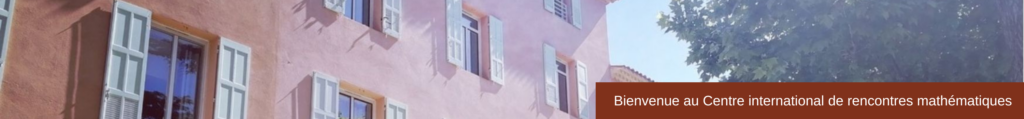Rechercher
Integrability and Randomness in Mathematical Physics
L’intégrabilité et l’aléatoire en physique mathématique
January-July 2019

 THEMES For 50 years, integrability has continuously staked claims in an ever-growing range of applications in mathematics and in physics. The modern theory of integrable systems grew up around the study of the Korteweg de Vries (KdV) equation, with origins in the seminal work of Kruskal and Zabusky about the recurrence behavior of solutions, the discovery of the Lax pair, multi-soliton solutions and infinite number of conservation laws. In later surprising connections, integrable systems like the KdV equation and the Toda lattice were proven to appear in fundamental combinatorial models, in random matrices and the geometry of moduli spaces. Discoveries from integrable systems have substantially influenced a number of areas of mathematical physics. Here is a short list of relevant examples: The generating function of Hurwitz numbers and various classes on moduli spaces coincide with tau-functions of integrable systems.   Partition functions of exactly solvable statistical models like the Ising model and the six-vertex model have been shown to be tau-functions of integrable equations  Random particle models appearing through connections to representation theory have an integrable structure, now referred to as integrable probability.   Local statistics of a large collection of matrix models and determinantal point processes in scaling limits (either in the bulk or at spectral edges) are related to integrable operators.   In general, integrability provides the route to an explicit description of behavior and phenomena that turns out to be ubiquitous in nonlinear systems. This statement is particularly true in the theory of nonlinear PDEs where the typical behavior of integrable PDEs is canonical far beyond the integrable examples.The central ambition of the programme will be to bring together mathematicians and physicists that work broadly in the field of integrability and randomness and to exchange and foster ideas and learn about new results and techniques. We expect that the themes of the semester will be, by its very nature, intertwined with a very wide swath of mathematical and scientific domains:​Mathematics: mathematical physics, integrable systems, probability, PDE, number theory, algebraic combinatorics. Physics: interacting particle systems and their interpretations in terms of interface growth models and directed polymers provide key predictions within non-equilibrium statistical mechanics. Random matrices are a basic tool, arising in various areas including statistical mechanics, spin-glasses, and quantum dynamics. LAUREATES CHAIR > Prof. Tamara GravaTamara Grava lectures at SISSA, Italy  and at Bristol University in the UK. Her research interests focus on the theory of integrable systems in mathematical physics and applied mathematics, and in particular on highly oscillatory Hamiltonian Partial Differential Equations, emergence of singular solutions from smooth initial data, numerical treatment ; Theta functions of Riemann surfaces and non-linear waves ; and on Riemann-Hilbert problems, special functions and orthogonal polynomials.  LOCAL PROJECT LEADER > Prof. Alexander Bufetov​Alexander Bufetov works for CNRS and is a member of the I2M department at Aix-Marseille University. His research interests lie in ergodic theory and dynamical systems. He is the Principal Investigator of the ERC Consolidator programme for the « IChaos » project. Bufetov was awarded the Prize of the Moscow Mathematical Society in 2005, the Sloan Fellowship and the Grant of the President of the Russian Federation in 2010 and the IUM-Simons Foundation Fellowship in 2011. Alexander Bufetov was also awarded the Sofia Kovalevskaya Prize from the Academy of Sciences of Russia on June 21st, 2016.

SCIENTIFIC PROGRAMME
Click on the blue button to find out detailed information on each event.
 Coulomb Gas, Integrability and Painlevé Equations (2105)Gaz de Coulomb, intégrabilité et équations de Painlevé11-15 March 2019
 Les mercredis mathématiques du CIRMA conference on the exceptional scientific contributions and the dramatic life of mathematician Andreï Kolmogorov (1903-1987) – ​Speaker: Alexander Bufetov (CNRS Aix-Marseille University)13 March 2019 at 14:30
 Integrability and Randomness in Mathematical Physics and Geometry (2104)L’intégrabilité et l’aléatoire en physique mathématique et en géométrie8-12 April 2019
 Integrability and Nonlinear Dispersive Equations (2106)Intégrabilité et équations dispersives non-linéaires24-28 June 2019
 Research in Pairs: Integrable Turbulence (2108)Turbulence intégrable6-17 May 2019
 Integrable Equation with Random Initial Data (2107)Equation intégrable et données initiales aléatoires10-21 June 2019
 List of guests in residence during this semester​Invités du semestre

POSTERS

VIDEOS